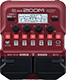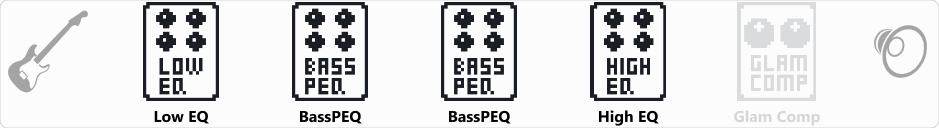# K.O.

Discussion in 'Zoom B1/B1X Four' started by Mod7, Sep 25, 2020.

1. K.O.Device: Zoom B1 Four
Firmware: 2.00

Name on device: K.O.
Optimized for: Guitar Amp

Effects chain:An effect that mimic the EHX's Knockout pedal which boost bass and/or treble while cuting the mids. Just set up the gains of each EQ. The Glam Comp can be kicked in if needed (slap for example).

Effect: "Low EQ" (Filter), active - "yes"
"Type" = SHELF
"Frequency" = 80
"Gain" = 9.0
"Volume" = 100

Effect: "BassPEQ" (Filter), active - "yes"
"Frequency" = 800Hz
"Q" = 1.0
"Gain" = -9.0
"Volume" = 100

Effect: "BassPEQ" (Filter), active - "yes"
"Frequency" = 2.0kHz
"Q" = 1.0
"Gain" = -6.0
"Volume" = 100

Effect: "High EQ" (Filter), active - "yes"
"Type" = SHELF
"Frequency" = 6400
"Gain" = 6.0
"Volume" = 100

Effect: "Glam Comp" (Dynamics), active - "no"
"Comp" = 50
"Shape" = 6
"Volume" = 25
"DryMx" = 60

Patch Volume: 100